# Algebra II : Graphing Systems of Equations and Inequalities

## Example Questions

### Example Question #1 : Graphing Systems Of Equations And Inequalities

This graph shows a solution at its intersection, the point (1,-1). This solution could be the solution for which of the following systems of equations?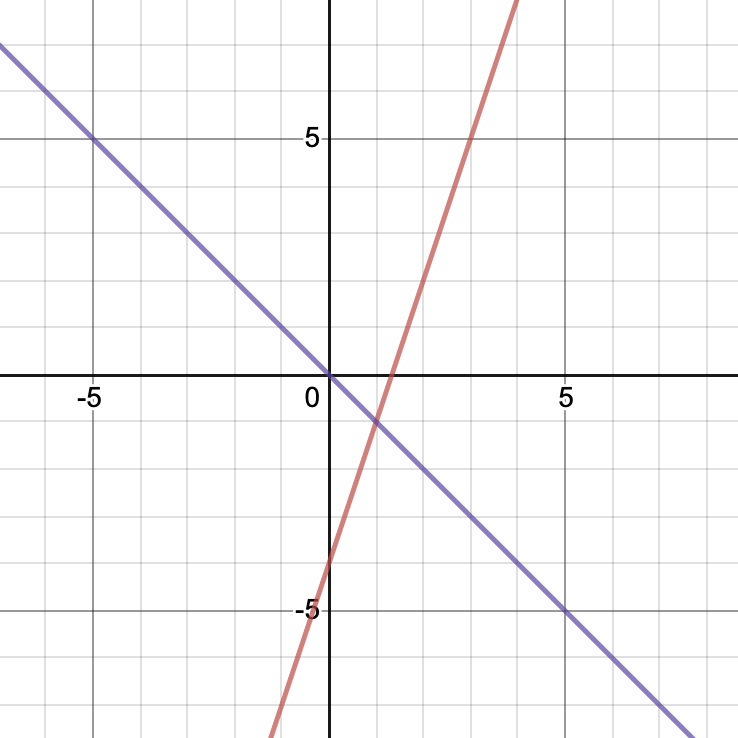Possible Answers: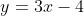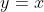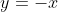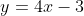Correct answer:Explanation:

Based on the graph, you can see that the purple line has the equationbased on the fact that it goes through the points (0,0) and (1,-1). The red line goes through the points (0,-4) and (1,-1). We can see that its slope is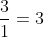and its y-intercept is at -4. Putting this into y=mx+b slope intercept form, we get. Therefore, this graph is the solution to the system of equationsAlf and Tanner are having a workout competition. Whoever completes the most workouts in a month gets $25 from the other. Alf has already completed 4 workouts, whereas Tanner has finished 2 of them. Going forward, Alf has committed to completing 2 workouts per week, and Tanner has committed to completing 3 per week. At some point soon, the two friends will have completed the same number of workouts. How many workouts will that be? Write a system of equations, graph them, and type the solution. Possible Answers: 2 workouts 8 workouts 5 workouts 10 workouts Correct answer: 8 workouts Explanation: A system of equations that models this, where a is the number of workouts Alf has completed and t is the number of workouts Tanner has completed is: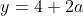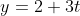Next, graph each of these equations: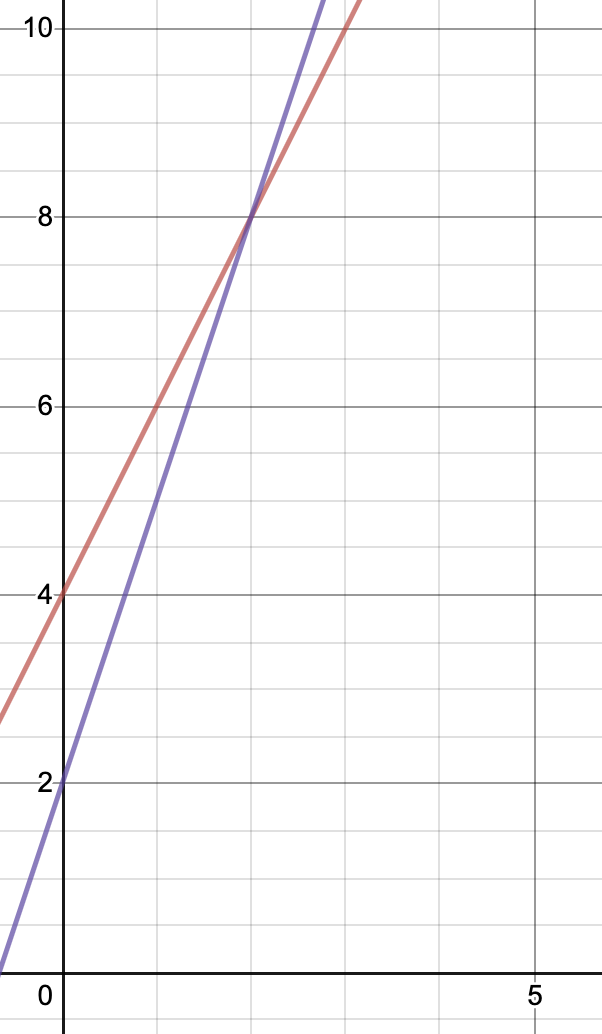Then, identify where the two lines meet each other. This is at (2,8). Therefore, after they each complete 8 workouts (in 2 weeks time) they will have completed the same number of workouts. ### Example Question #3 : Graphing Systems Of Equations And Inequalities This graph shows which of the following inequalities?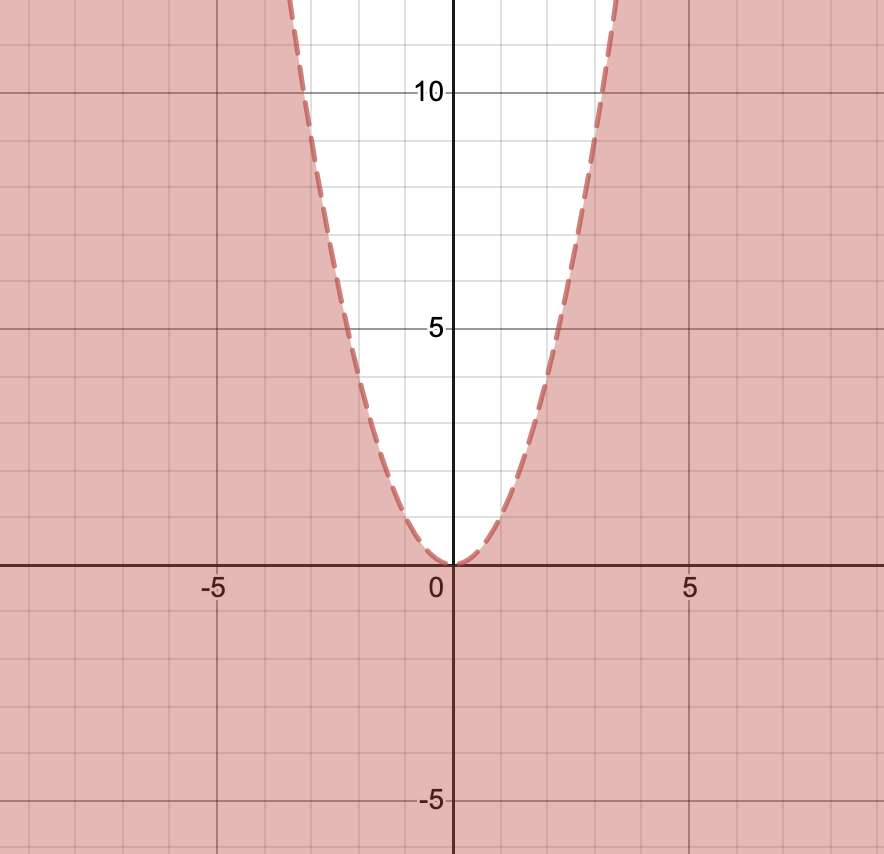Possible Answers: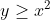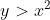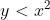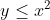Correct answer:Explanation: The function graphed is. We know that it is strictly less than because the line is dotted (not solid). We also know that y is less than because the portion of the graph below the function is shaded. ### Example Question #4 : Graphing Systems Of Equations And Inequalities Miles is a professional fitness instructor who will be teaching classes at a local gym. To get certified as an instructor, he spent a total of$400. Miles will be earning a base salary of $80 per month from the gym, plus an additional$20 for every class he teaches. If Miles teaches a certain number of classes during his first month as an instructor, he will earn back the amount he spent on certification. How many classes will that take?

Write a system of equations that models this situation, graph it, and type the solution.
Possible Answers: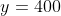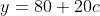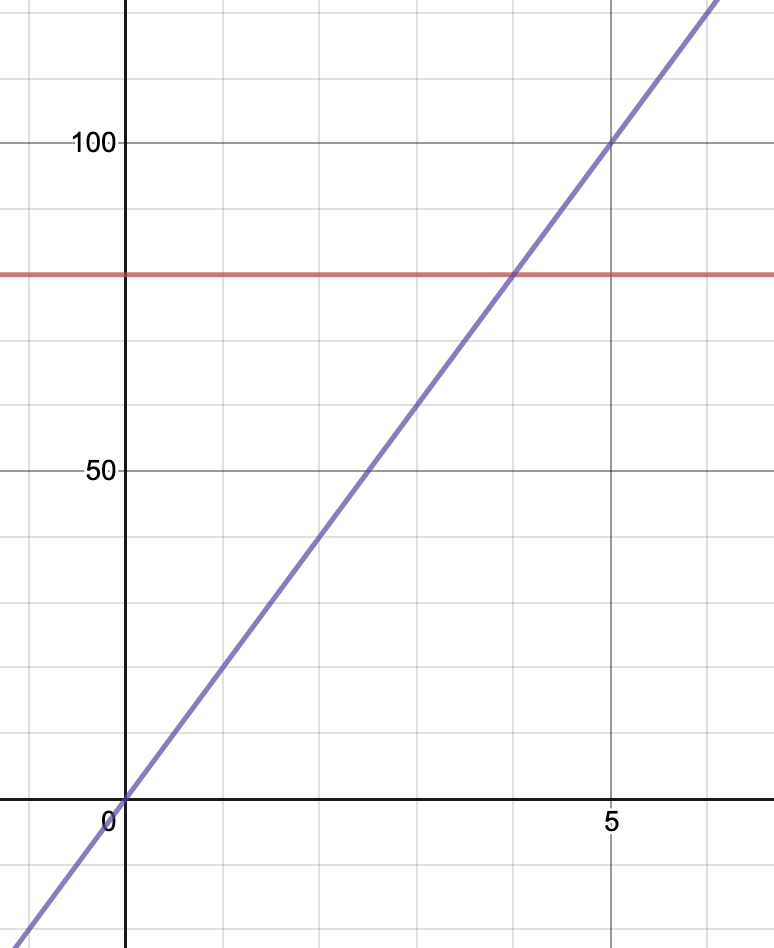16 classes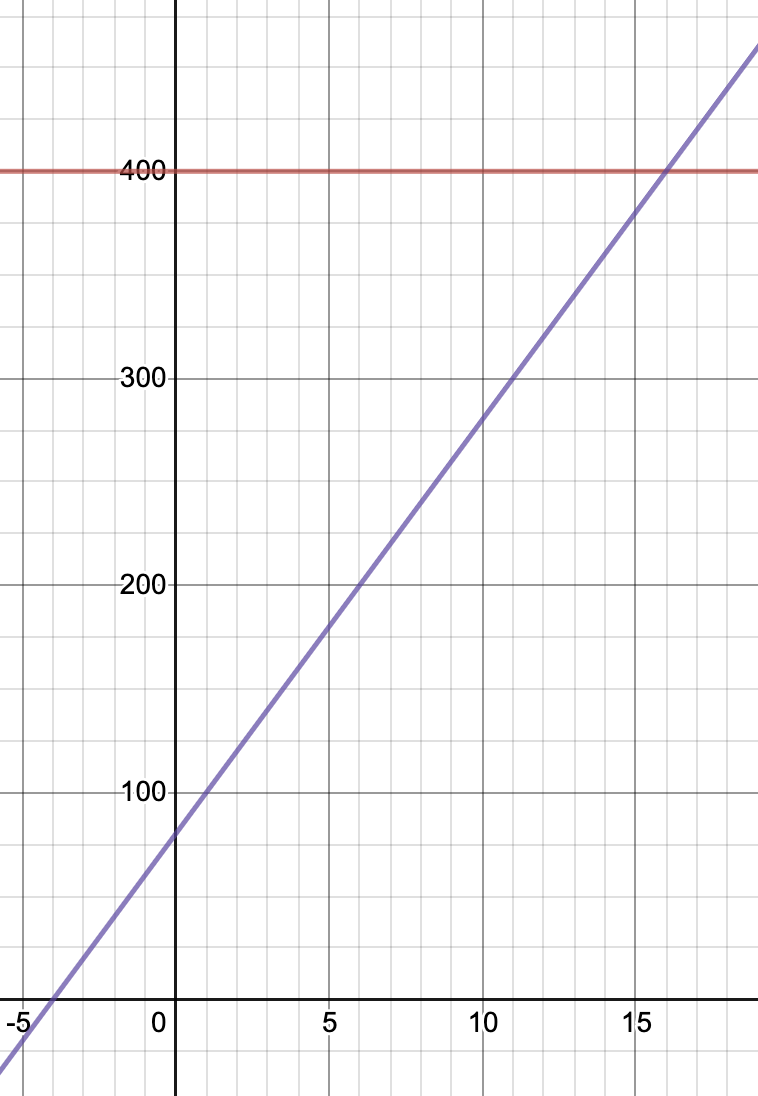16 classes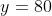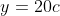4 classes4 classes

Correct answer:16 classes

Explanation:

To start, we want to write two equations that model this situation. Those equations are:(where c is the number of classes Miles teaches)

The graph of these two equations is below:Finally, the intersection of these two lines is associated with the total number of classes Miles needs to teach to break even. We can see that the intersection is at (16, 400). Therefore, after teaching 16 classes, Miles will have made $400 from the gym and broke even on his investment. ### Example Question #5 : Graphing Systems Of Equations And Inequalities Emeka is a professional fitness instructor who will be teaching classes at a local gym. To get certified as an instructor, he spent a total of$500. Emeka will be earning a base salary of $100 per month from the gym, plus an additional$20 for every class he teaches. If Emeka teaches a certain number of classes during his first month as an instructor, he will earn back the amount he spent on certification. How many classes will that take?

Write a system of equations that models this situation, graph it, and type the solution.
Possible Answers: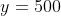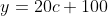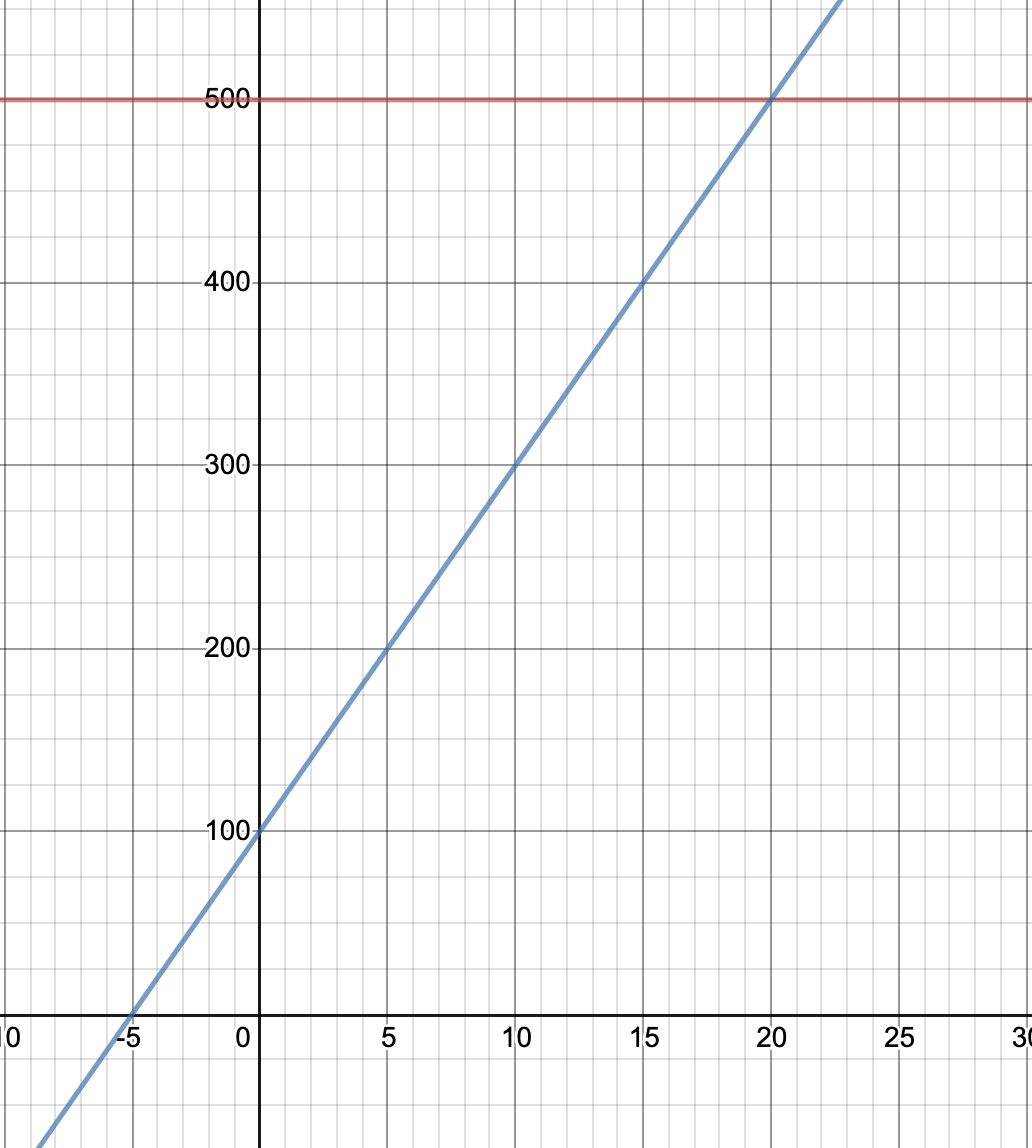500 classes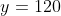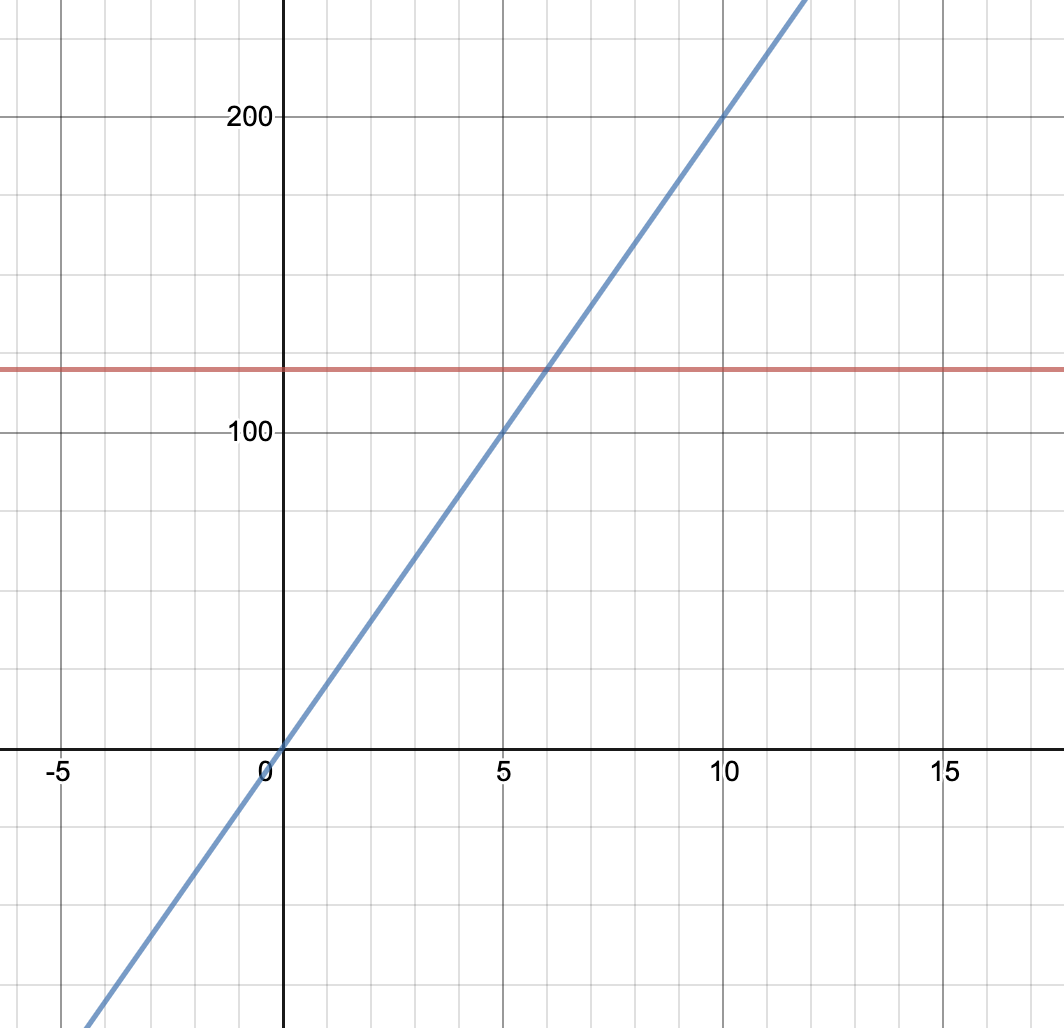120 classes6 classes20 classes

Correct answer:20 classes

Explanation:

To start, we want to write two equations that model this situation. Those equations are:(where c is the number of classes Emeka teaches)

The graph of these two equations is below:Finally, the intersection of these two lines is associated with the total number of classes Emeka needs to teach to break even. We can see that the intersection is at (20, 500). Therefore, after teaching 20 classes, Emeka will have made \$500 from the gym and broke even on his investment.

### Example Question #6 : Graphing Systems Of Equations And Inequalities

When graphed, the lines represented in the system of equations below will intersect in which quadrant?

3x - 2y = 0

4x+3y = -17

Possible Answers:

Quadrant IV

The lines will intersect on one of the axes, not in a quadrant.

Quadrant I

Quadrant III

Quadrant II

Correct answer:

Quadrant III

Explanation:

Recognize that the intersection of two lines occurs when the two lines meet at the same point - that is, where their x- and y-coordinates are the same. So you can find the point of intersection by simply solving the system. Here that is likely best done through the Elimination Method.  If you stack the equations:

3x - 2y = 0

4x+3y = -17

You should see that you have a negative y term in the first equation and a positive y term in the second. That means that if you can get the coefficients of y to be the same, you can sum the equations and eliminate the y-term altogether. To do that, multiply the top equation by 3 and the bottom equation by 2 so that your y-coefficients are each 6:

3(3x - 2y = 0) --> 9x - 6y = 0

2(4x + 3y = -17) --> 8x + 6y = -34

When you sum the equations, then, you arrive at:

17x = -34

Which means that x = -2.

Then plug x = -2 into one of the equations.  If you use the first, you'll get:

3(-2) - 2y = 0

Meaning that:

-6 - 2y = 0

-6=2y

y = -3

Since the point of intersection is (-2, -3), with both coordinates negative, the point will lie in Quadrant III.

### All Algebra II Resources